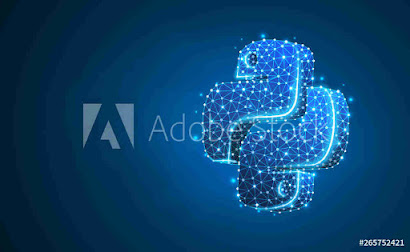Contents

# python recursion practice class 12

##### Q 1. what are the two cases required  in a recursive function.

ans. the two cases required  in a recursive function:-
(1)base case
(2)recursive case

### Q 2. it is necessary to have a base case in a recursive function?      why/why not.

ans. yes, it is necessary to have a base case in a recursive function. If there is no base case or the base case is not reachable, it leads to infinite recursion.

### Q 3. how can you stop/resolve an infinite recursion.

ans. the infinite recursion is stopped by providing a base case which must get executed, much be reachable for some argument value.

### Q 4. out of best-,average-,worst-case complexities, which one gives expected running time of an algorithm.

ans. average case compellability.

### Q 5. why are recursive function considered slower than iterative counterparts.

ans. recursive functions are slower as they involve multiple function calls. Additional function-call overheads make them consume more time.

### Q 6. what are base case and recursive case? what is their role in a recursive program?

ans. in a recursive solution ,the base cases are predetermined solutions for the simplest versions of the problem: if the given is a base case, no further computation is necessary to get the result.
the recursive case is the one that calls the function again with a new set of values. the recursive step is a set of rules that eventually reduces all versions of the problem to one of the base cases when applied repeatedly.

### Q 7. when does infinite recursion occur ?

ans. infinite recursion is when a recursive function executes itself again and again, endlessly. this happens when either the base case is missing or it is not reachable.

### Q 8. compare iteration and recursion.

ans. in, iteration, the code is executed repeatedly using the same memory space. that, the memory space allocated once, is used for each pass of the loop.

### Q 9. state one advantage and one disadvantage of using recursion over iteration.

ans. advantage:-recursion makes the code short and simple while iteration makes the code longer comparatively.
disadvantage :-recursion is slower than iteration due to overhead of multiple function calls and maintaining a stack for it.

### Q 10. figure out the error in the following recursive code of factorial: def   factorial(n): if n==0: return1 else: n*factorial(n-1) #_main_ print(factorial(4))

ans. the error in above code is that the recursive case is calling function factorial()with a changed value but returning the result of the call.
the correct version will be:-
def   factorial(n):
if n==0:
return1
else:
return n*factorial(-1)
#_main_
print(factorial(4))

### Q 11. why is the following code printing 1 endlessly. def   out_upto(n): i=1 if i>n: return print(i) i+=1 out_upto(i) out_upto(4)

ans. the above code is printing 1 endlessly because the base case i>n is never reachable. The reason is that value i becomes 1 for each call to function out_upto().

### Q 12. write recursive code to complete and print sum of square of n numbers. Value of n is passed as parameter.

ans.   def   sqsum(n):
if n==1:
return 1
return n*n + sqsum(n-1)
# _main_
n=int(input(“enter value of n:”))
print(sqsum(n))

### Q 13. write recursive code to compute greatest common divisor of two numbers.

ans.  def   gcd(a,b):
if(b==0):
return a
else:
return gcd(b,a%b)
n1=int(input(“enter first number:”))
n2=int(input(“enter second number:”))
d=gcd(n1,n2)
print(“gcd of”,n1,”and”,n2,”is:”,d)

### Q 14. program to show the use of recursion in calculation of power eg, ab.

ans.    def   power(a,b):
if b==0:
return 1
else:
return a*power(a,b-1)
#_main_
print(“enter only the positive numbers below”)
num=int(input(“enter base number:”))
p=int(input(“raised to the power of:”))
result=power(num,p)
print(num,”raised to the power of”,p,”is”,result)

### Q 15. recursive function to print string in reverse order.

ans.  def  reverse(s):
if len(s)==1:
return s
else:
return(s[-1] + reverse(s[0:len(s)-1))
print(reverse(“abc”))
output
cba

### 1 Response

1. November 9, 2020

[…]       important question of using python libraries (4)recursion     notes of recursion    important question of recursion (5)idea of algorithmic efficiency      notes of algorithmic efficiency             […]